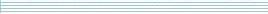| home      | people     | research     | publications    | seminars    | events     | contactCommunication Networks   | Systems Biology   | Hybrid Systems    | Machine Learning   | Dynamics & Interaction### Schools Mathematics Grand Challenge

Week eight's Puzzles

Problem 16:

The sixteenth problem was:

Three parties take part in a general election. Party A receives 40%, party B receives 30% and party C receives 30% of the votes cast in the election. Also:

(i) Of the people who voted for party A, 50% were women and 50% were men;
(ii) Of the people who voted for party B, 40% were women and 60% were men;
(iii) Of the people who voted for party C, 60% were women and 40% were men.

What percentage of the women who voted in the election voted for party B? Give your answer to the nearest percentage.

The solution was:

To work out the percentage of women who voted for party B, we need to figure out the total number of women and the total number of women who voted for party B.

Let V be the total number of people who voted. Then party A got 0.4V votes, party B got 0.3V votes and party C got 0.3V votes.

Now let's look at the women who voted for each party. Party A got votes from 0.5 x 0.4 V = 0.2V women. Party B got votes from 0.4 x 0.3 V = 0.12V women. Party C got votes from 0.6 x 0.3 V = 0.18V women.

So the total number of women who voted was 0.2V + 0.12V + 0.18V = 0.5V. The fraction that voted for party B was 0.12V / 0.5V = 0.24 or 24%.

Problem 17:

The seventeenth problem was:

There are 1,500 households in a housing estate. A certain number of the households have one car. Of the other households in the estate, 50% have two cars and the other 50% have no car. How many cars are in the housing estate in total?

The solution was:

Let T be the number of houses with 1 car. Then of (1500-T) houses there are half with no car and half with 2 cars. So, the total number of cars is

0 x (1500-T)/2 + 1 x T + 2 x (1500-T)/2 = 1500.

Problem 18:

The eighteenth problem was:

When you add up Jane's age, her mother's age and her father's age, their total age is 72. At the moment, Jane's father's age is 6 times Jane's age. When Jane's father's age is twice her age, the total age of the three of them will be twice what it is now. What is Jane's mother's age at the moment?

The solution was:

First let's write down all the information from the question.

We have 3 ages, Jane's age (J), her mother's age (M) and her father's age (F). When we add these up we get 72, so:

J + M + F = 72.

At the moment Jane's father is six times older than Jane, so:

6 J = F

and at some time in the future Jane's father will only be twice Jane's age. If this happens in N years, then

2(J + N) = F + N

and when this happens, the sum of all their ages will be twice what it is now:

(J+N + M+N + F+N) = 2(J + M + F).

Now we have all the information. The first and last equation look related, so let's rearrange the last equation as:

J + M + F + 3N = 2(J + M + F).

Now fill in that we know the sum of the ages is 72,

72 + 3N = 2(72)

we can use this to find out that N = 24. Now let's go back to the other equation with N in it and fill in 24:

2(J + 24) = F + 24

We can rearrange this to get 2J + 24 = F. But we also know F = 6J, so 2J + 24 = 6J, so J = 6 and F = 36. Finally, we know J + M + F = 72, so 6 + M + 36 = 72, so M = 30.